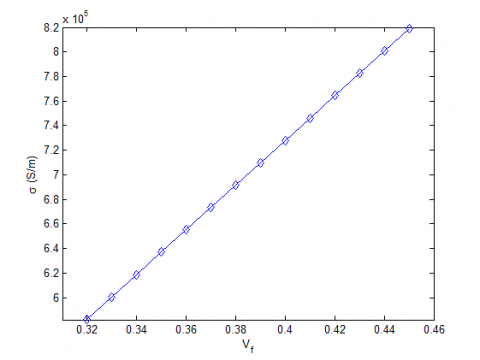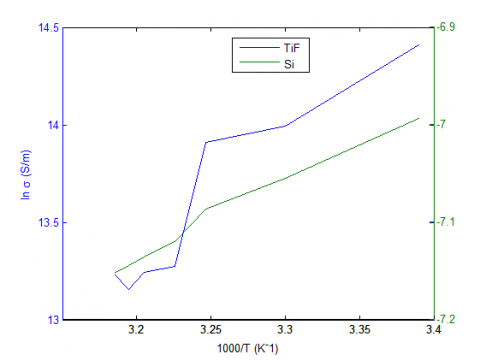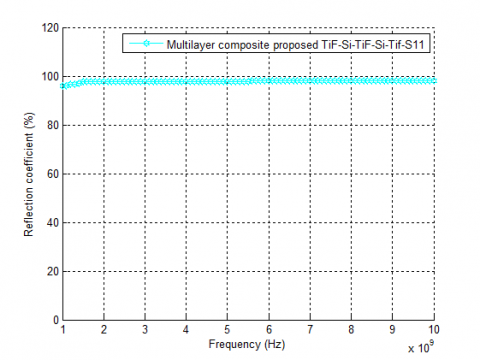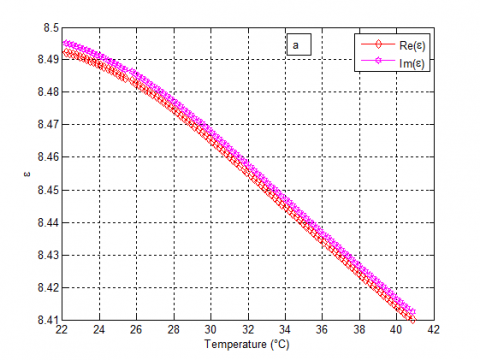# Effect of Human Body Temperature on New Multilayer Composite Shield in Pacemaker

Effect of Human Body Temperature on New Multilayer Composite Shield in Pacemaker

Laboratoire des Semi-conducteurs et Matériaux Fonctionnels, Université Amar Telidji de Laghouat, BP 37G, Laghouat 03000, Algeria

Laboratoire d’Analyse, de Commande des Systèmes d’Energie et Réseaux électriques, Université Amar Telidji de Laghouat, BP 37G, Laghouat 03000, Algeria

Corresponding Author Email:
t.merizgui@lagh-univ.dz
Page:
27-32
|
DOI:
https://doi.org/10.18280/rcma.290105
12 November 2018
|
Accepted:
15 January 2019
|
Published:
30 April 2019
| Citation

OPEN ACCESS

Abstract:

This paper presents a novel method for evaluating the effect of human body temperature on the electromagnetic shielding effectiveness (SE) of pacemaker. There are many sources of electromagnetic interference that interact with pacemakers: Cell phones may be considered responsible for electromagnetic interference EMI when they were held in the same part with the pacemaker; also, the magnetic resonance imaging makes a serious problem and must be avoided. In this paper, a new multilayer composite was prepared and built from alternating layers, the composite is reinforced by Titanium fibres and the dielectric layers made from the Silicon material, the dielectric layers are sandwiched between composites with Ti fibres symmetrically. Ti is the most widely used metal for pacemakers due to its high biocompatibility with the human body. Silicon has the advantages for being resistant material of temperature, lightweight, and its flexibility (bendable structure). The obtained simulation results prove the effectiveness of the proposed shield in the temperature range 37-41 °C, this is mainly due to the multi-reflection mechanism between the layers due to the high mismatched impedances.

Keywords:

EM shielding effectiveness, temperature, multilayer composite, pacemakers, titanium, silicon

1. Introduction

The electromagnetic interference become an important problem especially for the sensitive electronic devices such as Pacemakers (PM). The EMI shielding material is the main solution that reduce the emissions and improves the immunity of electronic equipment. Nowadays, the composite materials become an interesting material in many applications due to its lightweight; such as computation processes, military; and human health [1-3]. The metals are the most materials used in the shielding applications thanks to its high conductivity, but metals have few drawbacks such as prone to corrosion, are mainly by reflections, and heavyweights especially in the application where the mass should be low as low possible [4-10]. The efficient composite materials in shielding applications must be electrically conducting; the main responsible element for the conduction is the reinforcements in composite materials [24-26], several studies are reported on shielding materials like metallic coating, metallic fillers, and metallic fibers due to its excellent conductivity, permeability, and superficial skin depth .

The present paper has two aims; the first aim is to prepare a new multilayer composite to enhance the shielding effectiveness of composites, our proposed composite is made from Ti fibres dispersed in silicon (Si) matrix. The arrangement of conducting composite layer and Si layer are by alternating in the proposed multilayered structure. We suppose that our multi-layer structure contains a three-layered conductive composite of Titanium fibre (TiF) separated by a two-dielectric layer of silicon (Si) with 1 mm thick, to see how the multi-layered work against the electromagnetic interference (EMI). The three-layers has the same arrangement containing 60 fibres (TiF). With a conductivity σ=1.82*106 S/m, 0.035 mm radius, and 0.6 mm length embedded inside the dielectric layer (Si) a ɛ=11.9 and 0.5 mm thick. This composite structure (TiF/Si–Si–TiF/Si–Si–TiF/Si) can improve the electromagnetic interference EMI shielding effectiveness because the presence of layers permits to increase the reflection and multiple-reflection waves between the layers. The obtained results here prove the efficiency of our arrangement.

The second aim of this paper is to present a new prediction concerning our multi-layered structures for estimation the effect of human body temperature on the immunity of Pacemaker. The range of temperature here is between 37 to 41 °C which is the temperature variation of human bodies, the presented results show that the electrical conductivity is gradually decreases by increasing the temperature which affect directly on the total shielding effectiveness.

2. Model Setup

2.1 Multi-layer structure composite as EMI shielding materials

Electromagnetic shielding effectiveness EM SE is a measure of the material ability to attenuate EMI. The multilayer structure gives the desired objectives as the results displayed a decrease of absorption mechanism by the increase of reflection due to the high electrical conductivity which enhance the total shielding effectiveness [10-11]. Moreover, the proposed sample present a light weight. An incoming wave on a typical ribbon-shaped obstacle can be reflected, transmitted or absorbed. The absorption index is defined in Eq. (1) .

$A=1-R-T$ (1)

where R and T are the reflection and transmission power respectively, calculated based on the S parameters; it can be defined from the VNA as follows;

$R={{\left| {{S}_{11}} \right|}^{2}}={{\left| {{S}_{22}} \right|}^{2}},T={{\left| {{S}_{12}} \right|}^{2}}={{\left| {{S}_{21}} \right|}^{2}}$ (2)

${{S}_{11}}={{S}_{22}}=\frac{\Gamma \left( 1-{{T}^{2}} \right)}{1-{{\Gamma }^{2}}{{T}^{2}}},{{S}_{21}}={{S}_{12}}=\frac{T\left( 1-{{\Gamma }^{2}} \right)}{1-{{\Gamma }^{2}}{{T}^{2}}}$ (3)

where |Sij|2 represents the power reflection (i=j) and transmission (i≠j) from port i to port j.

Thus, the complex permittivity and the complex permeability can be expressed as

$\frac{{{\mu }_{r}}}{{{\varepsilon }_{r}}}=\left( \frac{1+\Gamma }{1-\Gamma } \right)={{C}_{1}},{{\mu }_{r}}{{\varepsilon }_{r}}=-{{\left\{ \left( \frac{c}{\omega t} \right)\ln \frac{1}{Z} \right\}}^{2}}={{C}_{2}}$ (4)

${{\varepsilon }_{r}}=\sqrt{\frac{{{C}_{1}}}{{{C}_{2}}}},{{\mu }_{r}}=\sqrt{{{C}_{1}}{{C}_{2}}}$ (5)

$\Gamma =\frac{Z-{{Z}_{0}}}{Z+{{Z}_{0}}}=\frac{\sqrt{\frac{{{\mu }_{r}}}{{{\varepsilon }_{r}}}}-1}{\sqrt{\frac{{{\mu }_{r}}}{{{\varepsilon }_{r}}}}+1}$ (6)

$Z={{e}^{-j\omega \sqrt{\mu \varepsilon d}}}={{e}^{\left( -j\left( \frac{\omega }{c} \right)\sqrt{{{\mu }_{r}}{{\varepsilon }_{r}}}d \right)}}$ (7)

where d represents the thickness of the proposed sample. Moreover, the SE depends on the type of source: plane wave, near electric field (E), or near magnetic field (H).

## 11111111111.jpgFigure 1. The proposed multilayer structure (TiF/Si-Si)

The general theoretical shielding effectiveness model of multi-layered materials in the far and near field regime vastly obtained in [12-14]. From the composite structure, we have proposed a new multi-layer structure with three layers (1, 3 and 5) display the same characteristic 60 fibres (TiF) with conductivity σ=1.82*106 S/m, at 0.035 mm radius dispersed in a dielectric layer (Si) a ɛ=11.9 with 0.8 mm thick. The three layers are separated via silicon (Si) at 1 mm thick, as shown in Figure 1.

The sum resistance of the composite can be calculated as:

$\frac{1}{{{R}_{C}}}=\frac{1}{{{R}_{f1}}}+\frac{1}{{{R}_{f2}}}+....+\frac{1}{{{R}_{f60}}}+\frac{1}{{{R}_{m}}}$ (8)

When considering the term $R=\frac{L}{A}\times \frac{1}{\sigma }$in Eq. (8), we got to manipulation:

${{\sigma }_{C}}={{\sigma }_{f}}\sum{\frac{{{A}_{fi}}}{{{A}_{C}}}+{{\sigma }_{m}}\frac{{{A}_{m}}}{{{A}_{C}}}}$ (9)

${{\sigma }_{C}}={{\sigma }_{f}}{{V}_{f}}+{{\sigma }_{m}}(1-{{V}_{f}})$ (10)

where σc is electrical conductivity of the composite (S/m), σmis electrical conductivity of the matrix (S/m), Vf is volume fraction of the Titanium fibres. Rm, Rc and Rf represent the resistance of the matrix, composite and fiber respectively, A is cross-section area of fuselage skin (m2). Various researchers [10, 15] appear that the fiber volume fraction is among the fundamental parameters in the determination of mechanical properties. Therefore, we want to determine its accurate estimate of fiber volume fraction is obtained according to ASTM D2584 as [15-16]:

${{V}_{f}}=\left[ {{\rho }_{m}}\times {{w}_{f}}/\left( {{\rho }_{m}}\times {{w}_{f}}+{{\rho }_{f}}\times {{w}_{m}} \right) \right]$ (11)

where Vf is the volume fraction of fibers, Wf, Wm is weight of fibers, and matrix, ρf, ρm is density of fibers and matrix respectively.

Figure (2) display the variation in electrical conductivity (S/m) versus volume fraction (Vf); where our results show a rise in the value of conductivity (σ) by growing the rate of volume fraction (Vf).

## 2.pngFigure 2. Variation in electrical conductivity vs volume fraction

2.2 The effect of temperature on the proposed multilayer composite

As shown in Figure 3 the temperature depends on electrical conductivity (S/m) of silicon matrix, and electrical conductivity (S/m) of fibers (Ti) as a function of 1000/T. The acquired curves decrease linearly for temperature increase, according to the Arrhenius law [17-18]:

${{\sigma }_{dc}}T={{A}_{0}}\exp -\left( \frac{{{E}_{a}}}{{{k}_{B}}T} \right)$ (12)

where A0 is the pre-exponential factor, Ea is the activation energy, and kB is the Boltzmann constant.

The effect of temperature on the electrical conductivity of Ti fibre, silicon matrix, and parameters of the electrical responses of the whole proposed composite sample are presented in Figure 3 and Table 1.

## 3.pngFigure 3. Temperature dependence of Ln (σT) for proposed composite

Table 1. The electrical conductivity obtained from the proposed sample

 Sample σ(S/m) at T=22°C σ(S/m) at T=37°C σ(S/m) at T=39°C σ(S/m) at T=40°C σ(S/m) at T=41°C (TiF) 1.8*106 5.8*105 5.6*105 5.61*105 5.59*105 (Si) 0.9*10-3 0.8*10-3 0.79*10-3 0.78*10-3 0.78*10-3
3. Results and Discussions

3.1 The effect of temperature on EMI shielding properties

Titanium (Ti) have better properties such as high conductivity, low cost, and light weight due to their enhanced contribute the better properties physical on a very large potential for many biomedical, electrical, structural, and electronic applications. The simulation results for the multi-reflection mechanism, and the total SE is obtained by the improving analytical model applied in . HFSS simulation (Version 13.0) was used to simulate the scattering parameters of the proposed multilayer composite in the frequency range of 1 to10 GHz.

Figure 4 illustrates the EMI multi-reflections of sandwiched structure composite of (TiF) in the frequency range 1 to 10 GHz. While the appearance of multi-reflection gain and the mismatch impedance increase the multiple internal reflections. The sum reflection into the structure and the results are indicate a higher reflectivity ratio due to their high electrical conductivity in a normal human body temperature (37 °C). Figure 5 shows the changes of total SE of the multilayer composite (TiF/Si–Si-TiF/Si–Si-TiF/Si) in the frequency range of 1–10 GHz. Evidently, the Ti fibers lead to the improvement of shielding effectiveness due to high reflection. Therefore, we can confirm that the decreases of multi-reflection at high temperature are mainly by the heat resistance of matrix (Si) because it provides the largest contribution to enhance the EMI SE of multilayer composite arrangements.

## 4.pngFigure 4. Multi-reflection mechanism of a composite material without (σH=1S/m)

## 5.pngFigure 5. Shielding effectiveness of a composite material without (σH=1S/m)

We notice a significant difference: the proposed multilayer composite with skin (biologic tissue) has a better performance because of the contribution to reverse electromagnetic waves in the near field due of (σH=1 S/m) compared to the results showed without the effect of a skin of the individual reflectivity. Whereas an impedance mismatch between the dielectric layer and conductive fibre (TiF) which increases multiple internal reflection inside the materials as shown in Figure 6.

Figure 7 shows the EMI shielding effectiveness of (TiF) in different degrees of temperature, the proposed five-layered structure (TiF–Si–TiF–Si–TiF) marks peak attenuation compared to other materials as shown in Table 1. As the wave progresses in the multilayer composite, reflection increases inside the materials. This result demonstrated that the proposed multilayer is more stable at a specific value of SE while there is a significant variation in the performance of the composite without taking into account the conductivity of the biologic tissue (σH= 1 S/m) as shown in Figure 8.

Figure 9 explain the magnitude S11of (TiF–Si–TiF–Si–TiF) at the frequency range 1 to 10 GHz, that multilayer composite presents high reflectivity around 96 and 100%.

## 6.pngFigure 6. Multi-reflection mechanism of a composite material with (σH=1S/m)

## 7.pngFigure 7. Shielding effectiveness of a composite material with (σH=1S/m)

## 8.pngFigure 8. Shielding effectiveness of a composite material with and without (σH=1S/m)

Table 2. EMI shielding performance of typical materials

 Material Layers Thickness of matrix Radius (fibre) SE (dB) Refs. µm mm µm mm Ti-Al2O3-Ti Third layer - 0.14 - - 120  Pani8.8/PU Third layer 100 - - - 40  GN-D-GN Third layer - 2 - - 36  GN- RGO(1)D-GN Third layer - 2 - - 32  GN- Fe3O4(1)D-GN Third layer - 2 - - 50  Pani8.8/PU Fifth layer 160 - - - 220  TiF/Si-Si Fifth layer - 0.5 - 0.035 115 This work

## 9.pngFigure 9. Simulation results of the parameter S11 in the proposed multilayer composite

3.2 EM parameter analysis of the proposed sample

The electromagnetic property of (TiF/Si) multi-layer composite is determined by complex permittivity (ε=ε'-jε"), and permeability (μ=μ'-jμ") . The real part of the electromagnetic parameters (ε', μ') is a measure of the magnitude of polarization occupation space in the proposed sample and display the ability for electric or magnetic potential storage, while the imaginary part (ε", μ") appear the potential loss .

Figure 10a shows the real part ɛ' and imaginary part ɛ'' of the multi-layer composite in the temperature range 37-41 °C at 10 GHz. Both the real and imaginary parts of permittivity progressively decrease while the temperature increase and takes the values about 8.49 at 22 °C, and 8.41 at 41 °C. These results indicate that the electric power storage ability of our sample has a great performance for acquired the improvement of the EMI shielding effectiveness.

Figure 10b present the real part µ' and imaginary part µ'' of the proposed multi-layer in the temperature range between 22 to 41 °C at 10 GHz. The real part of the permeability is decrease with increased temperature and changes from 0.2 at 22 °C, and marks a peak at 0.89 at 23.7 °C, then arrives at 0.32 at 41 °C. The imaginary part of the permeability increases progressively with the temperature; so the value changes from 0.3 at 22 °C, and 0.8 at 41 °C. The result obtained improve the EMI SE by an increasing of the multiple internal reflection mechanism.

## 10-1.png## 10.pngFigure 10. Simulation results of the electromagnetic parameter of multi-layer proposed

4. Conclusions

The impact of human body temperature on EMI shielding effectiveness of implanted electronic devices such as pacemakers is shown, and the performance of our smart arrangement composite (TiF) in a silicone matrix (Si) was presented. Multiple internal reflections mechanism caused by the big mismatch impedances helps to enhance the shielding effectiveness of multi-layers composite, as known the changes of human bodies temperature are between 37 to 41 °C. The results give a perfect EMI shielding effectiveness of the proposed structure, the reflection mechanism depends on the conductivity of proposed sample and especially the reflectivity of the biologic tissue, which contributed to the stability of this efficiency, in addition the thickness and the number of conductive layers and dielectric layers play an important role in the enhancement of SE. The (TiF/Si) were stacked in an accurate gradient of thickness to allows EM waves to penetrate deeply in the multilayer composite shield hence leads to a higher overall absorption power due to increase the multiple reflection mechanism.

Nomenclature
 A the absorption power T transmission power R reflection power d thickness A0 the pre-exponential factor Ea the activation energy KB Boltzmann constant. Vf the volume fraction Wf,m weight of fibers, and matrix T Temperature Greek symbols µr the complex permeability1 εr the complex permittivity σ electrical conductivity Subscripts EMI electromagnetic interference SE shielding effectiveness PM Pacemaker
References

 Fenical GG. (2006). New developments in shielding materials. 2006 IEEE Long Island Systems, Applications and Technology Conference. https://doi.org/10.1109/LISAT.2006.4302649

 Vas JV, Thomas MJ. (2018). Electromagnetic shielding effectiveness of layered polymer nanocomposites. IEEE Transactions on Electromagnetic Compatibility 60: 376-384. https://doi.org/10.1109/TEMC.2017.2719764

 Hayes DL, Carrillo RG, Findlay GK, Embrey M. (1996). State of the science: Pacemaker and defibrillator interference from wireless communication devices. Pacing Clin Electrophysiol 19(10): 1419-1430.

 Nishizawa S, Hashimoto O. (1999). Near field shielding effect for oval human model using high loss magnetic and dielectric materials. Tech. Rept. of IEICE, EMCJ99-14.

 Kurohwa S, Sato T, Hori K, Ishihara K. (1999). Effect of various shielding on mobile phone field intensity in human body. Proc. of Commun. Society Conf. of IEICE B-4-27.

 Micheli D, Apollo C, Pastore R, Barbera D, Morles RB, Marchetti M, Gradoni G, Primiani VM, Moglie F. (2012). Optimization of multilayer shields made of composite nanostructured materials. IEEE Trans. Electromagn. Compat. 54(1): 60-69. https://doi.org/10.1109/TEMC.2011.2171688

 Wang J, Fujiwara O, Nojima T. (2000). A Model for predicting electromagnetic interference of implanted cardiac pacemakers by mobile telephones. IEEE Trans. Microwave Theory Tech. 48(ll): 2121-2125. https://doi.org/10.1109/22.884203

 Ruoss HO, Jakobus U, Geisbusch L, Landstorfer FM. (1999). Efficient MoM–MMP hybrid method to investigate the coupling between electrodes of cardiac pacemakers and transmitting antennas. in 26th Gen. Assembly URSI, Toronto, ON, Canada 867.

 Lazzi G, Gandhi OP. (1998). On modeling and personal dosimetry of cellular telephone helical antennas with the FDTD code. IEEE Transactions on Antennas and Propagation 46(4): 525-529. https://doi.org/10.1109/8.664116

 Karteri I, Altun M, Gunes M. (2017). Electromagnetic interference shielding performance and electromagnetic properties of wood-plastic nanocomposite with graphene nanoplatelets. Journal of Materials Science Materials in Electronics 28(9): 6704-6711. https://doi.org/10.1007/s10854-017-6364-1

  Ji X, Wang Q, Yin F, Cui C, Ji P, Hao G. (2017). Fabrication and properties of novel porous CuAlMn shape memory alloys and polymer/CuAlMn composites. Composites: Part A 107(9): 21-30. https://doi.org/10.1016/j.compositesa.2017.12.013

 Nedjem Z, Seghier T, Hadjadj A. (2016). New multilayer arrangement of dielectric layers for enhancement of the magnetic shielding absorption at low frequency in the near field. Journal of Materials Science: Materials in Electronics 27(4): 3202-3208. https://doi.org/10.1007/s10854-015-4145-2

 Gaoui B, Hadjadj A, Kious M. (2016). Novel multilayer arrangement of conductive layers traps the electromagnetic interferences by multiple internal reflections at high frequency in the far field. Journal of Materials Science: Materials in Electronics 28(4): 3924-3930. https://doi.org/10.1007/s10854-016-6006-z

 Préault V, Corcolle R, Daniel L, Pichon L. (2014). Influence of skin effect on the effective shielding effectiveness of composite materials. Journal of Applied Physics 115(15): 154904. https://doi.org/10.1063/1.4871197

 Pan N. (1993). Theoretical determination of the optimal fiber volume fraction and layers fiber–matrix property compatibility of short fiber composites. Polymer Composites 14(2): 85-93. https://doi.org/10.1002/pc.750140202

 Gibson B, Cumiskey S, Christopher W. (2002). Compaction of fiber reinforcements. Polymer Composites 23(3): 307-318. https://doi.org/10.1002/pc.10433

 Wong KH, Pickering SJ, Rudd CD. (2010). Recycled carbon fibre reinforced polymer composite for electromagnetic interference shielding. Composites Part A: Applied Science and Manufacturing 41(9): 673-702. https://doi.org/10.1016/j.compositesa.2010.01.012

 Sharma S, Singh BP, Chauhan SS. (2018). Enhanced Thermomechanical and Electrical Properties of Multiwalled Carbon Nanotube Paper Reinforced Epoxy Laminar Composites. Composites: Part A 104(9): 129-138. https://doi.org/10.1016/j.compositesa.2017.10.023

 Micheli D, Apollo C, Pastore R, Barbera D, Morles RB, Marchetti M, Gradoni G, Primiani VM, Moglie F. (2012). Optimization of multilayer shields made of composite nanostructured materials. IEEE Transactions on Electromagnetic Compatibility 54(1): 60-69. https://doi.org/10.1109/TEMC.2011.2171688

 Preault V, Corcolle R, Daniel L, Pichon L. (2013). Effective permittivity of shielding composite materials for microwave frequencies. IEEE Transactions on Electromagnetic Compatibility 55(6): 1176-1178. https://doi.org/10.1109/TEMC.2013.2265173

 Nhan HN, Jean-Louis M, Jean-Luc W. (2006). Modeling of electromagnetic shielding effectiveness of multilayer conducting composites in the microwave band. 2006 First International Conference on Communications and Electronics, pp. 482-485. https://doi.org/10.1109/CCE.2006.350874

 Nhan HN. (2013). Characterisation and optimisation of the electromagnetic properties of conducting polymer composites in the microwave band. Int. J. Electr. Electron. Eng. Res. 3(1): 209-220.

 Song WL, Gong CC, Li H, Cheng XD, Chen M, Yuan X, Chen H, Yang Y, Fang D. (2017). Graphene-based sandwich structures for frequency selectable electromagnetic shielding. ACS Applied Materials & Interfaces 9(41): 36119-36129. https://doi.org/10.1021/acsami.7b08229

 Paulis F, Nisanci MH, Orlandi A, Koledintseva MY, Drewniak JL. (2014). Design of homogeneous and composite materials from shielding effectiveness specifications. IEEE Transactions on Electromagnetic Compatibility 56(2): 343-351. https://doi.org/10.1109/TEMC.2013.2280463

 Piersanti S, Paulis F, Orlandi A, Connor S, Liu Q, Archambeault B, Dixon P, Khoorrami M, Drewniak J. (2017). Near-field shielding performances of EMI noise suppression absorbers. IEEE Transactions on Electromagnetic Compatibility 59(2): 654-661. https://doi.org/10.1109/TEMC.2016.2626299

 Piersanti S, Orlandi A, Paulis F, Connor S, Khoorrami M, Dixon P, Archambeault B, Drewniak J. (2018). Near field shielding performances of absorbing materials for integrated circuits (IC) applications part I: Lateral excitation. IEEE Transactions on Electromagnetic Compatibility 60(1): 188-195. https://doi.org/10.1109/TEMC.2017.2726548 Hayes DL, Wang PJ, Reynolds DW, Estes M, Griffith JL, Steffens RA, Carlo GL, Findlay GK,

 Johnson CM. (1997). Interference with cardiac pacemakers by cellular telephones. The New England Journal of Medicine 336(21): 1473-1479. https://doi.org/10.1056/NEJM199705223362101